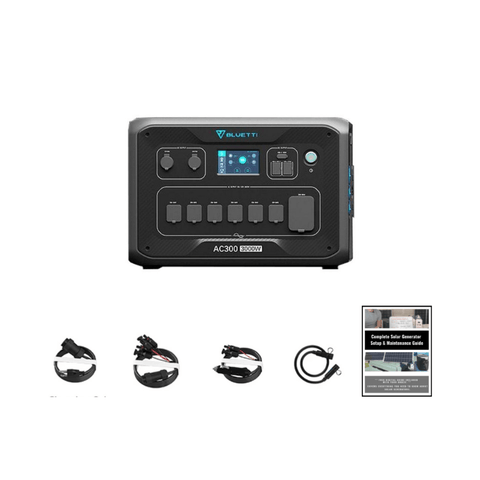Convert Milliamps to Amps (Easy to Follow Conversion Guide) - ShopSolar.com

# Convert Milliamps to Amps

The problem many new solar owners face is having to convert solar metrics like milliamps to amps. While this may seem straightforward, many solar guides tend to overcomplicate these equations and cause confusion.

Many solar converters and calculators exist online such as home amp calculators, RV amp calculators, and inverter amp draws calculators. However, we are going to be discussing the method of converting from milliamps to amps.

The good news is that our method doesn’t have any overcomplicated math and only relies on one simple formula that can be used to convert these two metrics quickly.

In solar panel systems, electric current is essential. Having the proper conversion equation is crucial to running a successful solar system and avoiding panel circuit damage.

## What’s the Difference Between Milliamps and Amps?

Before we get into the conversion, it’s important to understand the differences between milliamps and amps. This makes the conversion much easier.Let’s start by defining what an amp is.

An amp, also called an “ampere”, is a unit of electricity that measures how quickly an electrical current flow through a circuit. Amps are abbreviated as an ‘A’ in electrical equations.

A milliamp is simply one-thousandth of an ampere. Ultimately, it’s a smaller measurement of an amp that measures the same flow of current within a circuit.

The differences between these two terms are fundamental for converting from one to another and now that we know much more about them, we can show how the conversion is done.

### Milliamp to Amp Conversion

Converting between milliamps and amps is one of the easiest calculations to perform and you don’t need to be a maths boffin to execute it.

Firstly, you should always keep in mind how many amps are in a single milliamp. This will help you with the conversion calculation.

That being said, 1 milliamp is equal to 0.001 amps and 1 amp is equal to 1,000 milliamps. This is all you need to carry out your conversion.Here’s an example of it put into practice.

Let’s say you want to convert 20 milliamps to amps. There are two ways of doing this and they both have the same outcome.

First, you can take the 20 milliamps and divide it by 0.001, which we know is amp equivalent to 1 milliamp. This will give us 0.02 amps.

In the second method, you can take the 20 milliamps and divide it by 1,000. This will give us the same answer of 0.02 amps.

Additionally, if you want to work backward and figure out the milliamps from amps, then all you need to do is multiply the amp number by 1,000 to give you the milliamp equivalent.

That’s how easy it is to convert between milliamps and amps.

## Conclusion

Utilizing the sun's energy to power your home is one of the smartest decisions you make, especially if you’re trying to play your part in lowering your carbon footprint.

While the ecologically responsible lifestyle may initially be expensive, the wide plethora of benefits completely outweigh the initial price tag. These benefits range from reducing monthly utility bills to even gaining substantial income through solar tax incentives.

It may be difficult at first to convert solar electrical current, especially if you have to follow a detailed guide that employs tough techniques and calculations. Fortunately, the majority of solar conversion techniques are incredibly easy to understand and follow.

If you need any extra assistance choosing an electrical current converter, go to Shop Solar Kits. In addition to offering outstanding customer service, we can help you with any inquiries you may have about solar energy, including system setups incorporating solar-powered generators, such as our Bluetti AC300 Solar Generator.Hopefully, now you have a good understanding of solar conversions and how to easily perform the conversion between milliamps to amps. Perhaps next you can learn about converting volts to amps or maybe converting mWh to kWh.

So now that we know everything we need to know, let's make the smart decision and choose solar power.

Did You Find Our Blog Helpful? Then Consider Checking: